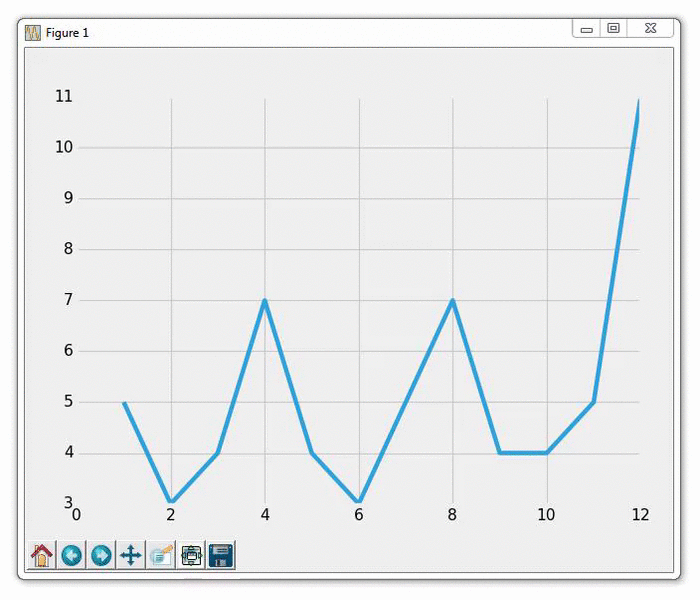# 第十六章 实时图表

``````import matplotlib.pyplot as plt
import matplotlib.animation as animation
from matplotlib import style
``````

``````style.use('fivethirtyeight')

fig = plt.figure()
``````

``````def animate(i):
lines = graph_data.split('\n')
xs = []
ys = []
for line in lines:
if len(line) > 1:
x, y = line.split(',')
xs.append(x)
ys.append(y)
ax1.clear()
ax1.plot(xs, ys)
``````

``````1,5
2,3
3,4
4,7
5,4
6,3
7,5
8,7
9,4
10,4
``````

``````ani = animation.FuncAnimation(fig, animate, interval=1000)
plt.show()
``````